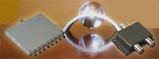# Low-Cost Power DividersJFW has introduced 2-way and 8-way, low-cost power dividers with a frequency range of 800 to 2400 MHz. Impedance is 50 Ohms nominal with an isolation of 20 dB minimum...

JFW has introduced 2-way and 8-way, low-cost power dividers with a frequency range of 800 to 2400 MHz. Impedance is 50 Ohms nominal with an isolation of 20 dB minimum.